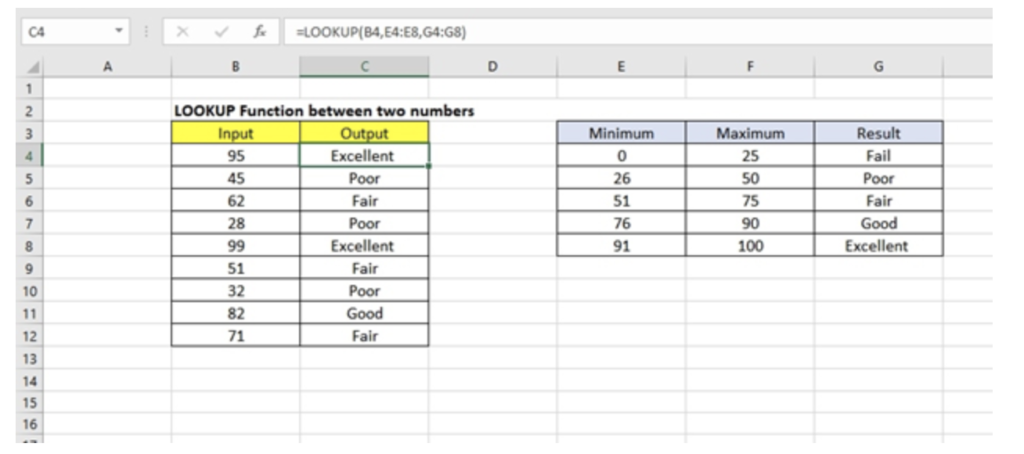Get instant live expert help with Excel or Google Sheets“My Excelchat expert helped me in less than 20 minutes, saving me what would have been 5 hours of work!”

#### Post your problem and you’ll get Expert help in seconds.

Your message must be at least 40 characters
Our professional Expert are available now. Your privacy is guaranteed.

# Lookup value between two numbers

## Generic FormulaLook Up Value Between Two Numbers

`=LOOKUP(B4,minimums,results)`

## Explanation

The LOOKUP function can be used to lookup the value between two numbers and deliver a commensurate result. To do this, the LOOKUP function and a sorted table will need to be used.

`=LOOKUP(lookup_value,lookup_vector,result_vector)`

Where:

• Lookup_value is the value that will be searched – which can either be a number, logical value or TRUE or FALSE, or a reference to a cell. (Required)
• Lookup_vector is the data to be searched. Usually, it is one-dimensional and must be sorted in ascending order. (Required)
• Result_vector is the list from which the value will be returned. It is also one-dimensional and must always be the same length as the lookup_vector (when supplied).

## How this formula works

When looking for the LOOKUP value between two numbers, the function performs a rough lookup in one range (lookup_value) and returns the value that corresponds in another range (result_vector).

To better understand this, let’s take a look at an example

## ExampleFigure 1. Lookup value between two numbers

The table above can be used to better understand the Lookup function. In our example above:

• Each value in the column labeled “Input” represents a lookup_value.
• Each value in the column labeled “Minimum” represents the lookup_vector.
• Each value in the column labeled “Result” represents the result_vector

For our example, we used only the minimum values since LOOKUP automatically matches the next small value once it can’t find a match for the value it is searching. So, if we were to rewrite our formula to match our example, it will be given as

`=LOOKUP(B4,E4:E8,G4:G8)`

## Notes

When looking up values between two numbers using the LOOKUP function, note the following:

• When an exact match of the lookup_value is matched in the lookup_vector, a commensurate value in the result_vector is returned.
• In the absence of a match, LOOKUP traverses the lookup vector until it finds a larger value, then it moves back to the previous row and returns a result.
• When the lookup_value is greater than the largest lookup_vector value, LOOKUP returns a result linked to the last lookup_vector value.
• Lookup_vector values must be sorted in ascending order.

Are you still looking for help with the VLOOKUP function? View our comprehensive round-up of VLOOKUP function tutorials here.

### Did this post not answer your question? Get a solution from connecting with the expert.Another blog reader asked this question today on Excelchat:
Solution examplesUse the Vlookup Function to complete the "employee" column of table 2. Use "job Id" from table 2 as your lookup_value(s) and table 1 as your reference.
Solved by C. H. in 16 minsIf a cell in another sheet is populated I need a vlookup done. If the cell is not populated I need the cell to return blank.
Solved by T. D. in 60 minsI am trying to make a chart that turns a week range red if nothing is entered in the range. If something is entered then I would like it to turn green. Please Help
Solved by E. U. in 43 minsI need a check box to show/hide an answer of an if function
Solved by Z. U. in 23 minsI need a formula to compare the data in two columns and then export the mismatched data in the 3rd column
Solved by S. Q. in 20 mins## Subscribe to Excelchat.coAnother blog reader asked this question today on Excelchat: